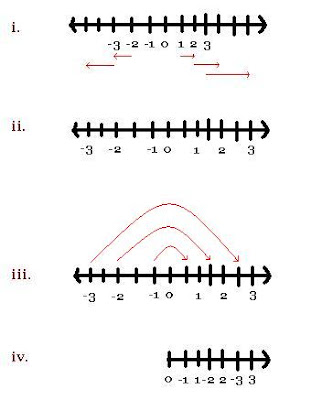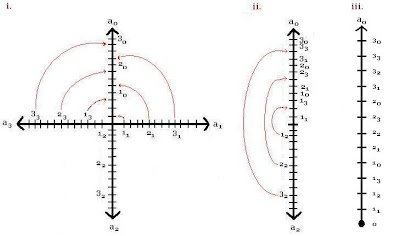## June 10, 2007

### Visual: Countable union of countable sets is countable

Mark Chu-Carroll at Good Math, Bad Math has had a few interesting posts lately on the Axiom of Choice and the equivalent Well-Ordering Principle, which says that any non-empty set of natural numbers (i.e., {1, 2, 3, ... }) has a least element. As I demonstrated awhile ago, this is used to justify the soundness of the proof technique called mathematical induction. Part of Mark's post focused on providing a well-ordering for the integers (i.e., {... -3, -2, -1, 0, 1, 2, 3, ...}), whereby the least element is 0 and the sequence proceeds thus: {0, -1, 1, -2, 2, ...}.

Verbal descriptions are well and good, but many find visual approaches more intuitive. For some odd reason, writers of higher math textbooks tend to shun visualizations, which is irresponsible since most great thinkers conceive images before writing them down in logical propositions and proofs. Maybe this antipathy is some silly relic of Platonic thinking that loathes the sense of sight, as it can mislead, as opposed to the faculties of disembodied logical reasoning. Only thing is: we're human, so most math ideas begin as pictures. We may flesh them out in chains of logical reasoning, but it is a fraud to present an idea only by showing logically that it is true, without any visual or other motivation beforehand.

In any case, what's going on with this well-ordering of the integers? Well, we start with the number line composed of the integers, "pinch" it at 0, and fold the negatives onto the same side as the positives, shoving the positive neighbors apart and shoe-horning each negative between two non-negative neighbors (e.g., -1 is stuck between 0 and 1, -2 is stuck between 1 and 2, and so on). To make it clear how the shoe-horning works so that no negative is accidentally fused on top of a non-negative, let's look at the following diagram:Imagine the individual integers as beads that rest on an infinitely long piece of string, which has tick marks where each integer is. To make room for the negative beads, we'll move each positive bead to the tick mark of its double -- that is, move 1 to 2, 2 to 4, 3 to 6, and in general move n to 2n, for positive n. Thus, the positive beads now rest only on the even tick marks on the positive side. Next, we move each negative bead to the tick mark of its double plus 1 -- that is, move (or keep) -1 to -1, move -2 to -3, -3 to -5, and in general move n to 2n + 1 for negative n. Now the negatives rest only on the odd tick marks on the negative side. We then send each negative n to its absolute value -- this where we rotate the set of negatives around the fulcrum 0, and since the positive odd spaces were cleared out in our first step, every negative bead has a place to fit in when folded onto the positive side. In this way, we get the well-ordering above: {0, -1, 1, -2, 2, ...}.

In reality, to well-order the integers, we could have "pinched" the number line at an arbitrary integer, not necessarily 0, and performed similar steps to zipper together the integers to the left of the fulcrum with those to the right. But using 0 makes the process clear.

In thinking up this visual, I came across another neat visual to show that the union of countably many countable sets is itself countable. Just now, we took 2 countably infinite sets -- the set of all positive integers and the set of all negative integers -- and formed a countable union. This is true because our beads occupy spaces whose tick marks are the natural numbers (and 0), and being able to bijectively map a set S to the natural numbers implies S is countable. But what if we wanted to do this for an arbitrarily large, though still countable, union of countable sets? This is why it's worth it to think in images, since we can instantly extend the visual analogy to this much larger, abstract case.

The problem now is how we zipper together this countably large union of countable sets? For simplicity, we'll consider each of these sets in the union as the natural numbers {1, 2, 3, ... }. I will frequently refer to the natural numbers both as just a countably infinite set, as well as an ordered sequence of terms (the obvious one -- (1, 2, 3, ...)). There is no potential for ambiguity since sets are not ordered while sequences are; this saves me from using roundabout ways of distinguishing what cannot be confounded.

Moving on, the trouble before was making room for every element of the set that we were attempting to shoe-horn in, and our solution was to assign one set to the even positives and the other set to the odd positives, so that no two distinct elements would ever overlap (and 0 would lie at the start, before the zippering). Suppose we have a third set to shoe-horn in -- on analogy with the simple case, we'll send the first set to {1, 4, 7, ...}, the second to {2, 5, 8, ...}, and the third to {3, 6, 9, ...}. Great, the trick works just as before!

In general, then, for the union of n countable sets, we partition the positive integers into n equivalence classes, where the members of a particular class are congruent to some integer a modulo n. That is, the members of a given class will have the same remainder when they are divided by n. In the example above, 1, 4, and 7 are all congruent to 1 mod 3 since they all leave a remainder of 1 when divided by 3. By assigning one countable set to the positives congruent to a mod n, another to the positives congruent to b mod n (where a =/= b), ad infinitum, we ensure that none of the elements overlap -- we can always shove the numbers aside to make room for the elements of yet another countable set. (A more formal proof concludes this post.) To help visualize this, remember before that we only had one set that we rotated around 0. In the general case, suppose that we had a countably large collection of arrows, along which the natural numbers were ordered (the numbers are only marked along one of the arrows to simplify the drawing, but all are so numbered):We pick one arrow as the starting point and order the rest in clockwise fashion. If there are n arrows, we label them a_0, a_1, a_2, ... a_(n - 1). We then assign the arrow labeled a_k to the equivalence class of positives congruent to k mod n (k ranging from 0 to n-1). So, our first arrow, a_0, is assigned to the positives congruent to 0 mod n -- that is, the multiples of n: {n, 2n, 3n, ... m*n} for m a positive integer, stipulating that none are mapped to 0 (we'll add it in at the end). We assign particular elements of a_0 to elements of {n, 2n, 3n, ...} in the obvious order-preserving way: the natural number m in a_0 is sent to the mth term of the sequence (n, 2n, ...) -- 1 is sent to 1*n, 2 to 2*n, etc.

Now that we've spread out the natural numbers along arrow a_0, we move clockwise to the next arrow a_1. As before, we assign a_1 to the set of positives congruent to 1 mod n -- (1, 1 + n, 1 + 2n, ...) -- so that the mth natural number in a_1 is sent to the mth term of the latter sequence. We continue around through the final arrow a_(n-1), assigning it to the set of positives congruent to (n-1) mod n, again sending the mth element of a_(n-1) to the mth term of the sequence (n - 1, 2n - 1, ...). Having thus spread out the natural numbers within each arrow to occupy only the positives congruent to a mod n, for all a distinct from each other and ranging from 0 to n - 1, we then rotate all n arrows around 0 so they coincide with our starting arrow a_0. Since equivalence classes don't overlap, we've ensured that no two numbers will accidentally collide with each other. We might as well add in 0 to the start of the sequence since that makes the "least element" easy to remember (this doesn't affect countability). Below is an example where we have 4 sets of natural numbers to zipper together. This is shown up through the first 3 elements from each arrow, although each arrow contains all natural numbers. To make the procedure easy to follow, I've added a subscript to each number to indicate which arrow it originated from (click to enlarge).Added: Note that not only is the union countable, but it's been ordered in a straightforward way.

More formal proof: We have bijectively mapped the elements of the union of n countable sets to the natural numbers (plus 0), which shows that this union is countable. Now add another arrow (i.e., another countable set, again assuming they're the natural numbers), so that there are now (n + 1) arrows. We translate the elements currently at non-zero multiples of n to non-zero multiples of (n + 1) in the obvious way -- the first element is sent to the first, and in general the mth element to the mth element between equivalence classes. Then we translate the elements currently at the positives congruent to (n - 1) mod n to the positives congruent to n mod (n + 1), again preserving the order. Continuing this way, we finally translate the elements currently at the positives congruent to 1 mod n to those congruent to 2 mod (n + 1). Having translated all elements from the case of only n arrows, we have now freed up spots at the set of positives congruent to 1 mod (n + 1), and we shoe-horn the elements of our new (n + 1)th arrow into these open spots in the order-preserving way.

So, assuming the procedure works for n arrows, it works for (n + 1) arrows. And we've already shown the procedure works for 2 arrows, so by induction we've proved that it works for any arbitrarily large, though countable, number of arrows. That is, the union of countably many countable sets is itself countable. (_)(_)

#### 1 comment:

1.You MUST enter a nickname with the "Name/URL" option if you're not signed in. We can't follow who is saying what if everyone is "Anonymous."